Home Practice
For learners and parents For teachers and schools
Textbooks
Full catalogue
Pricing SupportLog in

We think you are located in United States. Is this correct?

# 8.3 Le Chatelier's principle

## 8.3 Le Chatelier's principle (ESCNN)

Any factor that can affect the rate of either the forward or reverse reaction relative to the other can potentially affect the equilibrium position. The following factors can change the chemical equilibrium position of a reaction:

• concentration

• temperature

• pressure (for gaseous reactants)

It is important to understand what effect a change in one of these factors will have on a system that is in chemical equilibrium. However, performing an experiment every time to find out would waste a lot of time. Towards the end of the 1800s the French chemist Henry Louis Le Chatelier came up with principle to predict those effects.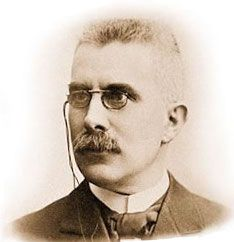Henry Louis Le Chatelier.

Le Chatelier's Principle helps to predict what effect a change in temperature, concentration or pressure will have on the position of the equilibrium in a chemical reaction. This is very important, particularly in industrial applications, where yields must be accurately predicted and maximised.

$$\color{darkgreen}{\Large\textbf{Le Chatelier's Principle}}$$

When an external stress (change in pressure, temperature or concentration) is applied to a system in chemical equilibrium, the equilibrium will change in such a way as to reduce the effect of the stress.

So if the concentration of one (or more) of the reactants or products is increased the equilibrium will shift to decrease the concentration. Or if the temperature is decreased the equilibrium will shift to increase the temperature by favouring the exothermic reaction. Le Chatelier's principle is that:

• If you change the $$\color{blue}{\textbf{concentration}}$$ of a reactant then the position of the equilibrium will shift to counteract that change.

• If you change the $$\color{red}{\text{temperature}}$$ of the reaction the equilibrium will shift to counteract that change.

• If you change the $$\color{orange}{\textbf{pressure}}$$ of the system the position of the equilibrium will shift to counteract that change.

Each of these concepts is discussed in detail in the following pages.

The following video gives an example of Le Chatelier's principle in action.

### The effect of concentration on equilibrium (ESCNP)

If the concentration of a substance is changed, the equilibrium will shift to minimise the effect of that change.

• If the concentration of a $$\color{blue}{\textbf{reactant}}$$ is increased the equilibrium will shift in the direction of the reaction that uses the reactants, so that the reactant concentration decreases. The forward reaction is favoured.

• The forward reaction is also favoured if the concentration of the $$\color{red}{\textbf{product}}$$ is decreased, so that more product is formed.

• If the concentration of a $$\color{blue}{\textbf{reactant}}$$ is decreased the equilibrium will shift in the direction of the reaction that produces the reactants, so that the reactant concentration increases. The reverse reaction is favoured.

• The reverse reaction is also favoured if the concentration of the $$\color{red}{\textbf{product}}$$ is increased, so that product is used.

For example, in the reaction between sulfur dioxide and oxygen to produce sulfur trioxide:

$\color{blue}{\text{2SO}_{2}\text{(g)}} + \color{blue}{\text{O}_{2}\text{(g)}} \rightleftharpoons \color{red}{\text{2SO}_{3}\text{(g)}}$

• If the $$\color{blue}{\text{SO}_{2}}$$ or $$\color{blue}{\text{O}_{2}}$$ concentration was increased:

• Le Chatelier's principle predicts that equilibrium will shift to decrease the concentration of reactants.

• Increasing the rate of the forward reaction will mean a decrease in reactants.

• So some of the sulfur dioxide or oxygen is used to produce sulfur trioxide.

• Equilibrium shifts to the right. That is, when a new equilibrium is reached (when the rate of forward and reverse reactions are equal again), there will be more product than before.

When the concentration of reactants is increased, the equilibrium shifts to the right and there will be more product than before. There will also be more reactants than before (more reactants were added). Once equilibrium has been reestablished (the rate of the forward and reverse reactions are equal again), $$\text{K}_{\text{c}}$$ will be the same as it was before the change to the system. This concept is explained in more detail later in this chapter.

• If the $$\color{blue}{\text{SO}_{2}}$$ or $$\color{blue}{\text{O}_{2}}$$ concentration was decreased:

• Le Chatelier's principle predicts that the equilibrium will shift to increase the concentration of reactants.

• Increasing the rate of the reverse reaction will mean an increase in reactants.

• So some sulfur trioxide would change back to sulfur dioxide and oxygen to restore equilibrium.

• Equilibrium shifts to the left. That is, when a new equilibrium is reached there will be less product than before.

• If [$$\color{red}{\text{SO}_{3}}$$] decreases:

• Le Chatelier's principle predicts that the equilibrium will shift to increase the concentration of products.

• Increasing the rate of the forward reaction will mean an increase in products.

• So some sulfur dioxide or oxygen is used to produce sulfur trioxide.

• Equilibrium shifts to the right. That is, when a new equilibrium is reached there will be more product than before.

• If [$$\color{red}{\text{SO}_{3}}$$] increases:

• Le Chatelier's principle predicts that the equilibrium will shift to decrease the concentration of products.

• Increasing the rate of the reverse reaction will mean a decrease in products.

• So some of the sulfur trioxide would change back to sulfur dioxide and oxygen to restore equilibrium.

• Equilibrium shifts to the left. That is, when a new equilibrium is reached there will be less product than before.

Although not required by CAPS the common-ion effect is a useful concept for the students to know if there is time.

Common-ion effect

In solutions the change in equilibrium position can come about due to the common-ion effect. The common-ion effect is where one substance releases ions (upon dissociating or dissolving) which are already present in the equilibrium reaction.

If solid sodium chloride is added to an aqueous solution and dissolves, the following dissociation occurs:

$\text{NaCl}(\text{s}) \to \text{Na}^{+}(\text{aq}) + \text{Cl}^{-}(\text{aq})$

If that solution contains the following equilibrium:

$\text{HCl}(\text{ℓ}) + \text{H}_{2}\text{O}(\text{ℓ}) \rightleftharpoons \text{Cl}^{-}(\text{aq}) + \text{H}_{3}\text{O}^{+}(\text{aq})$

The added $$\text{Cl}^{-}$$ ion (common-ion) interferes with the equilibrium by raising the concentration of the $$\text{Cl}^{-}$$ ion. According to Le Chatelier's principle the reverse reaction speeds up as it tries to reduce the effect of the added $$\text{Cl}^{-}$$. As a result the equilibrium position shifts to the left.

temp text

### The effect of temperature on equilibrium (ESCNQ)

If the temperature of a reaction mixture is changed, the equilibrium will shift to minimise that change.

• If the temperature is increased the equilibrium will shift to favour the reaction which will reduce the temperature. The endothermic reaction is favoured.

• If the temperature is decreased the equilibrium will shift to favour the reaction which will increase the temperature. The exothermic reaction is favoured.

For example the forward reaction shown below is exothermic (shown by the negative value for $$\Delta H$$). This means that the forward reaction, where nitrogen and hydrogen react to form ammonia, gives off heat, increasing the temperature (the forward reaction is exothermic). In the reverse reaction, where ammonia decomposes into hydrogen and nitrogen gas, heat is taken in by the reaction, cooling the vessel (the reverse reaction is endothermic).

Remember that heat is released during an exothermic reaction. It is a product of the reaction.

$$\color{blue}{\text{N}_{2}\text{(g)}} + \color{blue}{\text{3H}_{2}\text{(g)}} \rightleftharpoons {\color{red}{\text{2NH}_{3}\text{(g)}}} \qquad \Delta{H} = -92$$ $$\text{kJ}$$

• An increase in temperature:

• Favours the endothermic reaction because it takes in energy (cools the container).

• The reverse reaction is endothermic, so the reverse reaction is favoured.

• The yield of ammonia $$(\text{NH}_{3})$$ will decrease.

• A decrease in temperature:

• Favours the exothermic reaction because it releases energy (warms the container).

• The forward reaction is exothermic, so the forward reaction is favoured.

• The yield of $$\text{NH}_{3}$$ will increase.

In the informal experiment on Le Chatelier's principle, the solution should be purple to start. To achieve this $$\text{CoCl}_{2}$$ must be dissolved in ethanol and a few drops of water must be added. This solution is toxic, and all the usual laboratory precautions should be taken.

The hot water will make the solution a deep blue, the cold water will make the solution a pink/red colour. If necessary the test tube can be gently shaken to ensure mixing.

## Le Chatelier's principle

### Aim

To determine the effect of a change in concentration and temperature on chemical equilibrium

### Apparatus

• $$\text{0,2}$$ $$\text{mol·dm^{-3}}$$ purple $$\text{CoCl}_{2}$$ in ethanol solution, concentrated $$\text{HCl}$$, water

• test tube, tongs

• ice-bath, water-bath, hot-plate or bunsen burner

### Method

1. Place the water bath on the hot-plate and heat.

2. During each step observe and record the colour change that takes place.

3. Put $$\text{4}$$ – $$\text{5}$$ drops of $$\text{0,2}$$ $$\text{mol·dm^{-3}}$$ $$\text{CoCl}_{2}$$ solution into the test tube.

4. Add $$\text{10}$$ – $$\text{12}$$ drops of water.

5. Add $$\text{20}$$ – $$\text{25}$$ drops of concentrated $$\text{HCl}$$.

6. Place the test tube in the water-bath on the hot-plate (use tongs). Leave for $$\text{1}$$ – $$\text{2}$$ minutes. Record your observations.

7. Place the test tube in the ice-bath. Leave for $$\text{1}$$ – $$\text{2}$$ minutes. Record your observations.

The equation for the reaction that takes place is:

$\underset{\color{blue}{\text{blue}}}{\underbrace{{\color{blue}{{\text{CoCl}}_{4}^{2-}\text{(aq)}}}}} + 6{\text{H}}_{2}{\text{O(ℓ)}} \rightleftharpoons \underset{\color{red}{\text{pink}}}{\underbrace{{\color{red}{{\text{Co(H}}_{2}{\text{O)}}_{6}^{2+}\text{(aq)}}}}} + 4{\text{Cl}}^{-}\text{(aq)}$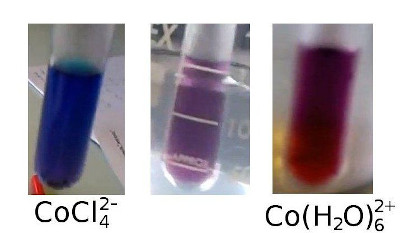### Results

Complete your observations in the table below, noting the colour changes that take place, and also indicating whether the concentration of each of the ions in solution increases or decreases.

 Original colour Final colour $${\color{blue}{{\text{[CoCl}}_{4}^{2-}{\text{]}}}}$$ $${\color{red}{{\text{[Co(H}}_{2}{\text{O)}}_{6}^{2+}{\text{]}}}}$$ $${\color{red}{{\text{[Cl}}^{-}{\text{]}}}}$$ Add $$\text{HCl}$$ Add $$\text{H}_{2}\text{O}$$ Increase temp Decrease temp

### Conclusions

• Use Le Chatelier's principle to explain the changes that you recorded in the table above.

• Draw a conclusion about the effect of a change in concentration of either the reactants or products on the equilibrium position.

• Also draw a conclusion about the effect of a change in temperature on the equilibrium position.

### The effect of pressure on equilibrium (ESCNR)

If the pressure of a gaseous reaction mixture is changed the equilibrium will shift to minimise that change.

• If the pressure is increased the equilibrium will shift to favour a decrease in pressure.

• If the pressure is decreased the equilibrium will shift to favour an increase in pressure.

When the volume of a system is decreased (and the temperature is constant), the pressure will increase. There are more collisions with the walls of the container. If there are fewer gas molecules there will be fewer collisions, and therefore lower pressure. The equilibrium will shift in a direction that reduces the number of gas molecules so that the pressure is also reduced. So, to predict in which direction the equilibrium will shift to change pressure you need to $$\color{darkgreen}{\text{look at the number of gas molecules in the balanced}}$$ $$\color{darkgreen}{\text{reactions}}$$.

$\text{p} \propto \dfrac{\text{T}}{\text{V}}$

That is, if the temperature remains constant, and the volume is increased, the pressure will decrease.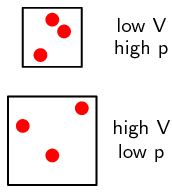Figure 8.2 shows a decrease in pressure by an increase in the volume, and an increase in pressure by a decrease in the volume.

For example, the equation for the reaction between nitrogen and hydrogen is shown below:

$$\color{blue}{\text{N}_{2}\text{(g)}} + \color{blue}{\text{3H}_{2}\text{(g)}} \rightleftharpoons \color{red}{\text{2NH}_{3}\text{(g)}}$$

The ratio in the balanced equation is $$1:3:2$$. That is, for every $$\text{1}$$ $$\text{molecule}$$ of $$\color{blue}{\text{N}_{2}\text{ gas}}$$ there are $$\text{3}$$ $$\text{molecules}$$ of $$\color{blue}{\text{H}_{2}\text{ gas}}$$ and $$\text{2}$$ $$\text{molecules}$$ of $$\color{red}{\text{NH}_{3}\text{ gas}}$$ (from the balanced equation). Therefore the ratio is $$\color{blue}{\textbf{4 molecules of reactant gas}}$$ to $$\color{red}{\textbf{2 molecules of product gas}}$$.

• An increase in pressure will:

• Favour the reaction that decreases the number of gas molecules.

• There are $$\color{red}{\textbf{fewer molecules of product gas}}$$ than reactant gas, so the forward reaction is favoured.

• The equilibrium will shift to the right and the yield of $$\color{red}{\textbf{NH}_{3}}$$ will increase.

• A decrease in pressure will:

• Favour the reaction that increases the number of gas molecules.

• There are $$\color{blue}{\textbf{more molecules of reactant gas}}$$, so the reverse reaction is favoured.

• The equilibrium will shift to the left and the yield of $$\color{red}{\textbf{NH}_{3}}$$ will decrease.

Consider illustration in Figure 8.2.Figure 8.2: (a) A decrease in the pressure of this reaction favours the reverse reaction (more gas molecules), the equilibrium shifts to the left. (b) An increase in the pressure of this reaction favours the forward reaction (fewer gas molecules), the equilibrium shifts to the right.

Figure 8.2 shows how changing the pressure of a system results in a shift in the equilibrium to counter that change. In the original system there are 12 molecules in total: $${\color{skyblue}{6{\text {H}}_{2}}} + {\color{blue}{2{\text{N}}_{2}}} \rightleftharpoons {\color{red}{4{\text{NH}}_{3}}}$$

If you decrease the pressure (shown by an increase in volume), the equilibrium will shift to increase the number of gas molecules. That shift is to the left and the number of $$\text{H}_{2}$$ and $$\text{N}_{2}$$ molecules will increase while the number of $$\text{NH}_{3}$$ molecules will decrease: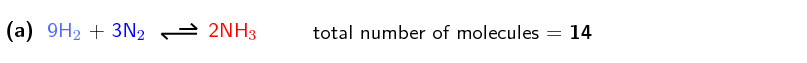If you increase the pressure (shown by a decrease in volume), the equilibrium will shift to decrease the number of gas molecules. That shift is to the right and the number of $$\text{H}_{2}$$ and $$\text{N}_{2}$$ molecules will decrease while the number of $$\text{NH}_{3}$$ molecules will increase: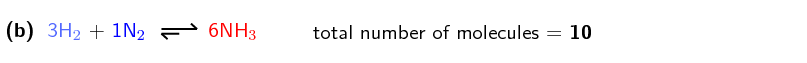Note that the total number of nitrogen and hydrogen atoms remains the same in all three situations. Equations (a) and (b) are not balanced equations.

Another example is the reaction between sulfur dioxide and oxygen:

$$\color{blue}{\text{2SO}_{2}\text{(g)}} + \color{blue}{\text{O}_{2}\text{(g)}} \rightleftharpoons \color{red}{\text{2SO}_{3}\text{(g)}}$$

In this reaction, $$\color{red}{\textbf{two molecules of product gas}}$$ are formed for every $$\color{blue}{\textbf{three molecules of}}$$ $$\color{blue}{\textbf{reactant gas}}$$.

• An increase in pressure will:

• Favour the reaction that decreases the number of gas molecules.

• There are $$\color{red}{\textbf{fewer molecules of product gas}}$$ than reactant gas, so the forward reaction is favoured.

• The equilibrium will shift to the right and the yield of $$\color{red}{\textbf{SO}_{3}}$$ will increase.

• A decrease in pressure will:

• Favour the reaction that increases the number of gas molecules.

• There are $$\color{blue}{\textbf{more molecules of reactant gas}}$$ than product gas, so the reverse reaction is favoured.

• The equilibrium will shift to the left and the yield of $$\color{red}{\textbf{SO}_{3}}$$ will decrease.

temp text

### The effect of a catalyst on equilibrium (ESCNS)

If a catalyst is added to a reaction, both the forward and reverse reaction rates will be increased. If both rates are increased then the concentrations of the reactants and products will remain the same. This means that a catalyst has no effect on the equilibrium position.

However, a catalyst will affect how quickly equilibrium is reached. This is very important in industry where the longer a process takes, the more money it costs. So if a catalyst reduces the amount of time it takes to form specific products, it also reduces the cost of production.

### Factors that influence the value of $$\text{K}_{\text{c}}$$ (ESCNT)

Concentration, pressure, and temperature all affect the equilibrium position of a reaction, and a catalyst affects reaction rates. However, only $$\color{red}{\textbf{temperature}}$$ affects the value of $$\text{K}_{\text{c}}$$.

• Changing $$\color{blue}{\text{concentration}}$$:

• Changing the concentration of a reactant or product results in one of the reactions (forward or reverse) being favoured.

• This change in reaction rate minimises the effect of the change and restores the concentration ratio between reactants and products. There will just be more reactants and products.

• $$\color{blue}{\text{K}_{\text{c}}}$$ $$\color{blue}{\text{will remain the same}}$$.

• Changing $$\color{orange}{\text{pressure}}$$:

• Changing the pressure of the system will change the ratio between the reactant and product concentrations.

• The equilibrium then shifts to minimise the effect of the change and restores the ratio between reactant and product concentrations.

• $$\color{orange}{\text{K}_{\text{c}}} \color{orange}{\text{ will remain the same}}$$.

• Adding a $$\color{purple}{\text{catalyst}}$$ to the system:

• Both the forward and reverse reactions rates are increased.

• Therefore the ratio between reactant and product concentrations will remain the same.

• $$\color{purple}{\text{K}_{\text{c}}}\color{purple}{\text{ will remain the same}}$$.

• Changing $$\color{red}{\text{temperature}}$$:

• Changing the temperature will favour either the endothermic or exothermic reaction.

• The ratio between the concentration of the reactants and products will change.

• $$\color{red}{\text{K}_{\text{c}}}$$ $$\color{red}{\text{will change}}$$.

So make sure that when comparing $$\text{K}_{\text{c}}$$ values for different reactions, the different reactions took place at the same temperature.

### Using Le Chatelier's Principle (ESCNV)

When a system is in chemical equilibrium, and there has been a change in conditions (e.g. concentration, pressure, temperature) the following steps are suggested:

1. Identify the disturbance or stress on the system.

$$\color{blue}{\text{For example, there is an }\textbf{increase}\text{ in the concentration of reactant}}$$.

2. Use Le Chatelier's principle to decide how the system will respond.

$$\color{blue}{\text{Le Chatelier predicts a shift to }\textbf{decrease}\text{ the concentration of reactant}}$$.

3. Look at the given equation and decide whether the rate of the forward reaction or the rate of the reverse reaction is increased. State the shift in equilibrium.

$$\color{blue}{\text{The }\textbf{forward reaction will be favoured}\text{. The equilibrium will shift }\textbf{to the right}}$$.

4. Where appropriate, link equilibrium shift to any observed change in the system.

$$\color{blue}{\text{This might result in a }\textbf{colour change}}$$.

## Worked example 6: Using Le Chatelier's principle

Table salt is added to the (purple) solution in equilibrium:

$$\underset{\color{blue}{\text{blue}}}{\underbrace{{\color{blue}{{\text{CoCl}}_{4}^{2-}}}}} + 6{\text{H}}_{2}{\text{O}} \rightleftharpoons \underset{\color{red}{\text{pink}}}{\underbrace{{\color{red}{{\text{Co(H}}_{2}{\text{O)}}_{6}^{2+}}}}} + 4{\text{Cl}}^{-}$$

1. Use Le Chatelier's principle to predict the change in equilibrium position.

2. What would be observed?

### Identify the disturbance or stress on the system

Adding $$\text{NaCl}$$ produces $$\text{Na}^{+}$$ ions and $$\text{Cl}^{-}$$ ions as the salt dissolves. Looking at the given equilibrium $$\text{Cl}^{-}$$ is in the equation and the disturbance is the increase in concentration of the $$\text{Cl}^{-}$$ ion.

### Use Le Chatelier's principle to decide how the system will respond

By Le Chatelier's principle, the equilibrium position will shift to reduce the concentration of $$\text{Cl}^{-}$$ ions.

### Decide whether the rate of the forward reaction or the rate of the reverse reaction is increased and state the resulting shift in equilibrium

The reverse reaction uses $$\text{Cl}^{-}$$ ions and hence the rate of the reverse reaction will increase. The reverse reaction is favoured and the equilibrium will shift to the left.

### What would the colour change be due to this equilibrium shift?

The solution will appear more blue as more blue $$\text{CoCl}_{4}^{2-}$$ ions are formed.

#### Graphs and Le Chatelier's principle

Graphs can be used to represent data about equilibrium reactions. The following are some points to keep in mind when presented with a graph.

1. Identify the type of graph by looking at the label on the y-axis. You will find either:

1. a rate-time graph

2. a mole-time or concentration-time graph

2. For rate-time graphs, when the rate for the forward reaction and the rate for the reverse reaction are equal, the system is in equilibrium.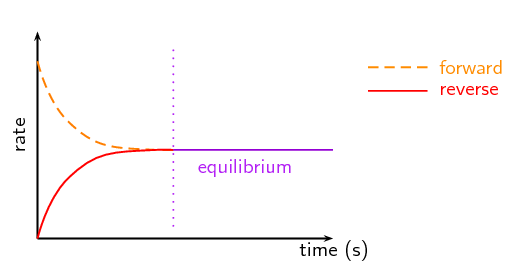3. For concentration-time graphs or mole-time graphs equilibrium occurs where the concentration or number of moles of the reactants and products are constant. These values need not be equal to one another.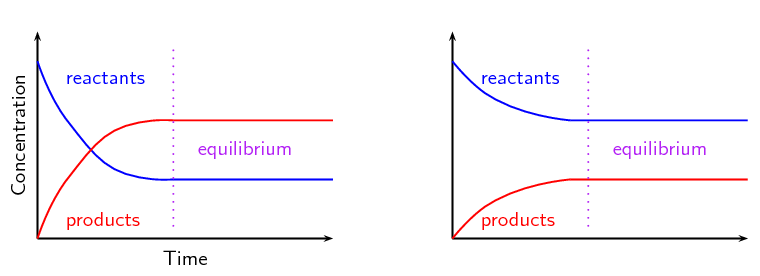4. When calculating $$\text{K}_{\text{c}}$$ make sure you only take values from the sections of the graph where the y-value is constant. $$\text{K}_{\text{c}}$$ can only be calculated when the system is in equilibrium.

Rate-time graphs

• A change in concentration of a substance will favour the reaction that counteracts that change.

• An increase in concentration of either the reactants or the products will result in both the forward and reverse reaction rates being higher once equilibrium is re-established.

An increase in reactant concentration will favour the forward reaction. The forward reaction rate will increase sharply, and then gradually decrease until equilibrium is re-established. At the same time the reverse reaction rate will gradually increase.

An increase in product concentration will favour the reverse reaction. The reverse reaction rate will increase sharply, and then gradually decrease until equilibrium is re-established. At the same time the forward reaction rate will gradually increase.

• A decrease in concentration of either the reactants or the products will result in both the forward and reverse reaction rates being lower once equilibrium is re-established.

A decrease in reactant concentration will favour the reverse reaction. The forward reaction rate will decrease sharply, and then gradually increase until equilibrium is re-established. At the same time the reverse reaction rate will gradually decrease.

A decrease in product concentration will favour the forward reaction. The reverse reaction rate will decrease sharply, and then gradually increase until equilibrium is re-established. At the same time the forward reaction rate will gradually decrease.

• A change in pressure of a reaction will favour the reaction that counteracts that change.

• An increase in pressure will sharply increase both the forward and reverse reaction rates.

The reaction that will reduce the pressure (fewer gas particles) will be favoured and will initially increase more. That reaction rate will then decrease gradually until equilibrium is re-established.

The other reaction will initially increase less, and then gradually continue to increase until equilibrium is re-established.

• A decrease in pressure will sharply decrease both the forward and reverse reaction rates.

The reaction that will increase the pressure (more gas particles) will be favoured and will initially decrease less. That reaction rate will then continue to decrease gradually until equilibrium is re-established.

The other reaction will initially decrease more, and then gradually increase until equilibrium is re-established.

• A change in temperature of a reaction will favour the reaction that counteracts that change.

• An increase in temperature will sharply increase both the forward and reverse reaction rates.

The reaction that will decrease the temperature (the endothermic reaction) will be favoured and will initially increase more. That reaction rate will then decrease gradually until equilibrium is re-established.

The other reaction will initially increase less, and then gradually continue to increase until equilibrium is re-established.

• A decrease in temperature will sharply decrease both the forward and reverse reaction rates.

The reaction that will increase the temperature (the exothermic reaction) will be favoured and will initially decrease less. That reaction rate will then continue to decrease gradually until equilibrium is re-established.

The other reaction will initially decrease more, and then gradually increase until equilibrium is re-established.

• The addition of a catalyst will favour both the forward and reverse reactions by the same amount.

## Worked example 7: Rate-time graphs

For the reaction $$2\text{AB}(\text{g})$$ $$\rightleftharpoons$$ $$2\text{A}(\text{g}) + \text{B}_{2}(\text{g})$$, $$\Delta$$H = $$\text{26}$$ $$\text{kJ}$$

the following graph can be plotted: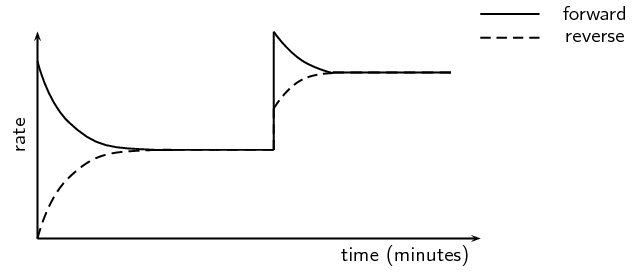What stress has occurred in this system? Label the graph with what is happening at each stage.

### Check the axes so that you know what the variables are on this graph

The axes are labelled rate and time. Therefore this is a rate-time graph.

### Are the rates both affected in the same way or is one rate increased and the other decreased when the stress is applied?

Both rates are affected in the same way (increased) therefore the stress must be a catalyst or a change in temperature.

(A change in pressure or concentration would favour one reaction direction only)

### Are both rates affected equally?

No, the forward rate is increased more than the reverse rate. Therefore the stress must be a change in temperature.

(A catalyst would increase both rates equally)

### Was the temperature increased or decreased? (Use Le Chatelier's principle)

The forward reaction is endothermic ($$\Delta$$H is positive). The forward reaction was favoured more than the reverse reaction. An increase in temperature will favour the reaction that cools the reaction vessel (the endothermic reaction). Therefore the stress must have been an increase in temperature.

### Label what is occuring at each stage on the graph## Worked example 8: Rate-time graphs

What is responsible for the change at t = $$\text{10}$$ $$\text{minutes}$$ in the graph below?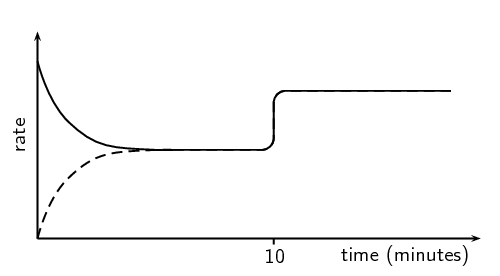### Check the axes so that you know what the variables are on this graph

The axes are labelled rate and time. Therefore this is a rate-time graph.

### Are the rates both affected in the same way or is one rate increased and the other decreased when the stress is applied?

Both rates are affected in the same way (increased) therefore the stress must be a catalyst or a change in temperature.

(A change in pressure or concentration would favour one reaction direction only)

### Are both rates affected equally?

Yes, both rates are increased by the same amount.

### What was responsible for the change at t = $$\text{10}$$ $$\text{minutes}$$?

The addition of a catalyst (a change in temperature would affect both rates, but unequally).

Concentration-time and mole-time graphs

• A change in concentration of a substance would appear as a sharp increase or decrease in the concentration or number of moles of that substance and a gradual change in the other substances.

• A change in temperature would affect both the forward and reverse reactions. However, one reaction would be affected more than the other. So the reactants and products would be affected gradually, in the opposite direction (one increased, the other decreased).

• A change in pressure of the reaction would cause a sharp increase or decrease in all the reactants and products. For an increase in pressure, if the forward reaction is then favoured the reactant concentrations will decrease, and if the reverse reaction is then favoured the product concentrations will decrease.

• The addition of a catalyst would increase both the forward and reverse reaction rates, meaning the equilibrium is reached faster. So if the reaction is already at equilibrium there will be no effect on a concentration-time or moles-time graph.

## Worked example 9: Concentration-time graphs

Consider the following chemical equation and graph and answer the questions that follow.

$\text{CO}(\text{g}) + \text{Cl}_{2}(\text{g}) \rightleftharpoons \text{COCl}_{2}(\text{g})$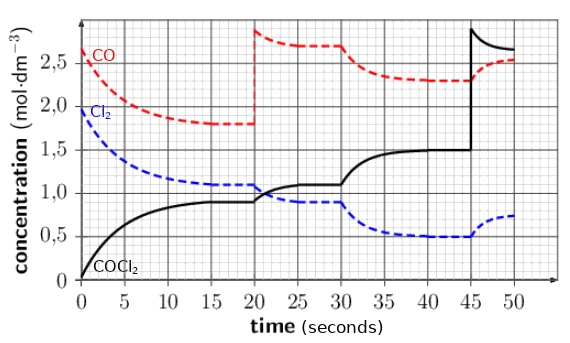1. How much time was necessary for the system to reach equilibrium for the first time?

2. How do the rates of the forward and reverse reactions compare at the following times:

• $$t = \text{5}$$ $$\text{s}$$

• $$t = \text{17}$$ $$\text{s}$$

• $$t = \text{23}$$ $$\text{s}$$

3. Determine the equilibrium constants for the system at $$t = \text{17 s}$$, $$\text{27 s}$$, and $$\text{42 s}$$.

4. What happens at $$t = \text{20 s}$$? Explain your answer by referring to Le Chatelier's principle.

5. What effect does the stress at $$t = \text{20 s}$$ have on $$\text{K}_{\text{c}}$$?

### Check the axes so that you know what the variables are on this graph

The axes are labelled concentration and time. Therefore this is a concentration-time graph.

### How much time was necessary for the system to reach equilibrium for the first time?

The concentration of all three compounds becomes constant at t = $$\text{15}$$ $$\text{s}$$. This means that the reaction has reached equilibrium.

### How can you determine which rate is faster from concentration-time graphs?

If the concentrations of the reactants are steadily decreasing and the concentrations of the products are steadily increasing then the forward reaction is using reactants faster than the reverse reaction is using products. The rate of the forward reaction must be faster than the rate of the reverse reaction.

If the concentrations of the reactants are steadily increasing and the concentrations of the products are steadily decreasing then the forward reaction must be using reactants slower than the reverse reaction is using products. The rate of the forward reaction must be slower than the rate of the reverse reaction.

If the concentrations of the reactants and products are constant, the forward reaction must be using reactants at the same rate as the reverse reaction is using products. The rate of the forward reaction must be equal to the rate of the reverse reaction (the system is in equilibrium).

### Compare the forward and reverse reaction rates at $$t = \text{5} \text{ s}$$, $$t = \text{17} \text{ s}$$ and $$t = \text{23} \text{ s}$$

At $$t = \text{5} \text{ s}$$ and at $$t = \text{23} \text{ s}$$ the concentrations of the reactants are decreasing and the concentration of the product is increasing. Therefore the rate of the forward reaction is faster than the rate of the reverse reaction.

At $$t = \text{17} \text{s}$$ the concentrations of the reactants and products are constant (unchanging). Therefore the reaction is in equilibrium and the rate of the forward reaction equals the rate of the reverse reaction.

### What are the concentrations of $$\text{CO}$$, $$\text{Cl}_{2}$$ and $$\text{COCl}_{2}$$ at $$t = \text{17} \text{ s}$$, $$t = \text{27 s}$$ and $$t = \text{42 s}$$?

Reading off the graph you can see that:

 Equilibrium concentration $$(\text{mol·dm^{-3}})$$ Substance $$t = \text{17 s}$$ $$t = \text{27 s}$$ $$t = \text{42 s}$$ $$\text{CO}$$ $$\text{1,8}$$ $$\text{2,7}$$ $$\text{2,3}$$ $$\text{Cl}_{2}$$ $$\text{1,1}$$ $$\text{0,9}$$ $$\text{0,5}$$ $$\text{COCl}_{2}$$ $$\text{0,9}$$ $$\text{1,1}$$ $$\text{1,5}$$

### Write an expression for the equilibrium constant of this reaction and calculate $$\text{K}_{\text{c}}$$.

$$\text{K}_{\text{c}} = \dfrac{\text{[COCl}_{2}{\text{]}}}{\text{[CO][Cl}_{2}{\text{]}}}$$

At $$t = \text{17 s}$$: $$\;\text{K}_{\text{c}} = \dfrac{\text{0,9}}{(\text{1,8})(\text{1,1})} =$$ $$\text{0,45}$$

At $$t = \text{27 s}$$: $$\;\text{K}_{\text{c}} = \dfrac{\text{1,1}}{(\text{2,7})(\text{0,9})} =$$ $$\text{0,45}$$

At $$t = \text{42 s}$$: $$\;\text{K}_{\text{c}} = \dfrac{\text{1,5}}{(\text{2,3})(\text{0,5})} =$$ $$\text{1,30}$$

Note that $$\text{K}_{\text{c}}$$ is different at $$\text{42 s}$$. This means that the stress at $$\text{30 s}$$ must have been a change in temperature.

### What happens at $$t = \text{20 s}$$?

The concentration of $$\text{CO}$$ increases sharply. The concentrations of the other compounds do not change dramatically. Therefore $$\text{CO}$$ must have been added to the system.

After this addition of $$\text{CO}$$ there is a shift to reduce the amount of $$\text{CO}$$, that is in the forward direction. Therefore the concentration of the product increases while the concentrations of the reactants decrease.

### What effect will this have on $$\text{K}_{\text{c}}$$?

Only a change in temperature affects $$\text{K}_{\text{c}}$$, therefore this will have no effect on $$\text{K}_{\text{c}}$$.

## Worked example 10: Concentration-time graphs

Consider the following chemical equilibrium and graph and answer the questions that follow.

$$\text{H}_{2}(\text{g}) + \text{I}_{2}(\text{g})$$ $$\rightleftharpoons$$ $$2\text{HI}(\text{g})$$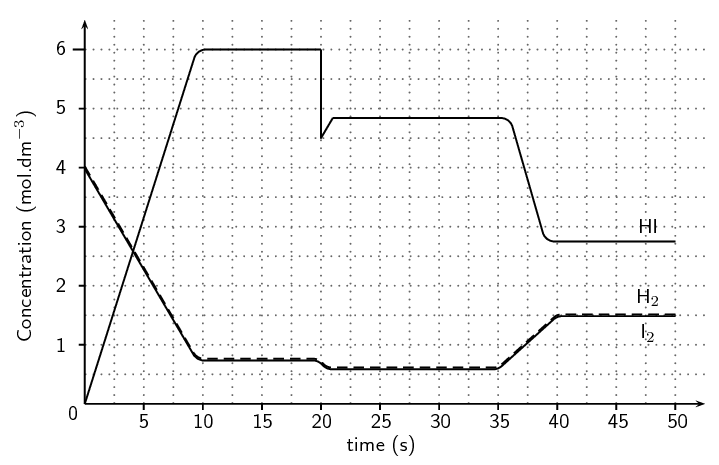1. After how many seconds does the system reach equilibrium?

2. Calculate the value of the equilibrium constant.

3. Explain what happened at t = $$\text{20}$$ $$\text{s}$$.

4. If the change at t = $$\text{35}$$ $$\text{s}$$ is due to an increase in temperature, is the reaction exothermic or endothermic? Explain your answer.

### Check the axes so that you know what the variables are on this graph

The axes are labelled concentration and time. Therefore this is a concentration-time graph.

### After how many seconds does the system reach equilibrium?

The concentration of all three compounds becomes constant at t = $$\text{10}$$ $$\text{s}$$. This means that the system takes $$\text{10}$$ $$\text{s}$$ to reach equilibrium.

### What are the concentrations of $$\text{H}_{2}$$, $$\text{I}_{2}$$, and $$\text{HI}$$ at t = $$\text{10}$$ $$\text{s}$$, $$\text{25}$$ $$\text{s}$$, and $$\text{45}$$ $$\text{s}$$?

Reading off the graph you can see that at $$\text{10}$$ $$\text{s}$$:

$$[\text{H}_{2}]$$ and $$[\text{I}_{2}]$$ = $$\text{0,75}$$ $$\text{mol·dm^{-3}}$$, $$[\text{HI}]$$ = $$\text{6,0}$$ $$\text{mol·dm^{-3}}$$

At $$\text{25}$$ $$\text{s}$$:

$$[\text{H}_{2}]$$ and $$[\text{I}_{2}]$$ = $$\text{0,6}$$ $$\text{mol·dm^{-3}}$$, $$[\text{HI}]$$ = $$\text{4,8}$$ $$\text{mol·dm^{-3}}$$

At $$\text{45}$$ $$\text{s}$$:

$$[\text{H}_{2}]$$ and $$[\text{I}_{2}]$$ = $$\text{1,5}$$ $$\text{mol·dm^{-3}}$$, $$[\text{HI}]$$ = $$\text{2,75}$$ $$\text{mol·dm^{-3}}$$

### Write an expression for the equilibrium constant of this reaction and calculate $$\text{K}_{\text{c}}$$.

$$\text{K}_{\text{c}} = \dfrac{\text{[HI]}^{2}}{\text{[H}_{2}{\text{][I}_{2}{\text{]}}}}$$

At t = $$\text{10}$$ $$\text{s}$$:

$$\text{K}_{\text{c}} = \dfrac{\text{6,0}^{2}}{(\text{0,75})(\text{0,75})} =$$ $$\text{64,0}$$

At t = $$\text{25}$$ $$\text{s}$$:

$$\text{K}_{\text{c}} = \dfrac{\text{4,8}^{2}}{(\text{0,6})(\text{0,6})} =$$ $$\text{64,0}$$

At t = $$\text{45}$$ $$\text{s}$$:

$$\text{K}_{\text{c}} = \dfrac{\text{2,75}^{2}}{(\text{1,5})(\text{1,5})} =$$ $$\text{3,4}$$

The different $$\text{K}_{\text{c}}$$ at $$\text{45}$$ $$\text{s}$$ means that the event at t = $$\text{35}$$ $$\text{s}$$ must be a change in temperature.

### Explain what happened at t = $$\text{20}$$ $$\text{s}$$?

The concentration of $$\text{HI}$$ decreases sharply, as a result there is a slight decrease in the concentration of $$\text{H}_{2}$$ and $$\text{I}_{2}$$. Therefore $$\text{HI}$$ must have been removed from the system.

After this there is a shift to increase the amount of $$\text{HI}$$, that is in the forward direction.

### If the change at t = $$\text{35}$$ $$\text{s}$$ is due to an increase in temperature, is the reaction exothermic or endothermic? Explain your answer.

An increase in temperature caused the concentration of the product to decrease and the concentrations of the reactants to increase. This means that the reverse reaction has been favoured.

An increase in temperature will favour the reaction that takes heat in and cools the reaction vessel (endothermic). Therefore the reverse reaction must be endothermic and the forward reaction must be exothermic. The reaction is exothermic.

### Summary (ESCNW)

The following rules will help in predicting the changes that take place in equilibrium reactions:

• If the concentration of a reactant (on the left) is increased, then some of it must be used to form the products (on the right) for equilibrium to be maintained. The equilibrium position will shift to the right. $$\text{K}_{\text{c}}$$ is unchanged.

• If the concentration of a reactant (on the left) is decreased, then some of the products (on the right) must be used to form reactants for equilibrium to be maintained. The equilibrium position will shift to the left. $$\text{K}_{\text{c}}$$ is unchanged.

• If the forward reaction is endothermic, then an increase in temperature will favour this reaction and the product yield and $$\text{K}_{\text{c}}$$ will increase. A decrease in temperature will decrease product yield and likewise decrease $$\text{K}_{\text{c}}$$.

• If the forward reaction is exothermic, then an decrease in temperature will favour this reaction and the product yield and $$\text{K}_{\text{c}}$$ will increase. An increase in temperature will decrease product yield and likewise decrease $$\text{K}_{\text{c}}$$.

• Increasing the pressure favours the reaction that will decrease the number of gas molecules. This is shown in the balanced chemical equation. This rule applies in reactions with one or more gaseous reactants or products. $$\text{K}_{\text{c}}$$ is unchanged.

• Decreasing the pressure favours the the reaction that will increase the number of gas molecules. This rule applies in reactions with one or more gaseous reactants or products. $$\text{K}_{\text{c}}$$ is unchanged.

• A catalyst does not affect the equilibrium position of a reaction. It only influences the rate of the reaction, in other words, how quickly equilibrium is reached.

## Worked example 11: Chemical equilibrium

$$2\text{NO}(\text{g}) + \text{O}_{2}(\text{g})$$ $$\rightleftharpoons$$ $$2\text{NO}_{2}(\text{g})$$ $$\quad \Delta{H} < 0$$

How will:

1. the reverse reaction

2. the equilibrium position be affected by:

1. A decrease in temperature?

2. The addition of a catalyst?

3. The addition of more $$\text{NO}_{2}$$ gas?

1. The reverse reaction:

1. The forward reaction is exothermic ($$\Delta{H} < 0$$) so the reverse reaction must be endothermic. A decrease in temperature will cause the equilibrium to shift to favour the exothermic reaction. Therefore the reverse reaction rate will decrease sharply, and then gradually increase until equilibrium is re-established.

2. The addition of a catalyst will speed up both the forward and reverse reactions.

3. $$\text{NO}_{2}$$ is a product. Therefore the addition of more $$\text{NO}_{2}$$ will increase the rate of the formation of reactants. The rate of the reverse reaction will therefore increase sharply, and then gradually decrease until equilibrium is re-established.

2. The equilibrium position:

1. A decrease in temperature will favour the exothermic reaction and the forward reaction is exothermic. Therefore the equilibrium position will shift to the right.

2. The addition of a catalyst will have no effect on the equilibrium position as both the forward and reverse reactions rates would be increased equally.

3. The addition of more $$\text{NO}_{2}$$ will favour the formation of the reactants and so the equilibrium will shift to the left.

## Worked example 12: Graphs of equilibrium

Study the graph and answer the questions that follow: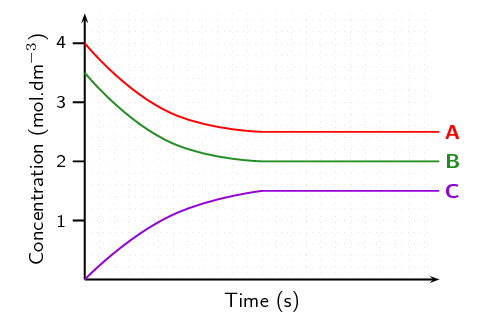1. Does the equilibrium favour the reactants or the products?

2. Determine the value of $$\text{K}_{\text{c}}$$ if the coefficients of the balanced equation all equal 1.

3. The forward reaction has $$\Delta{H} > 0$$. What effect will an increase in temperature have on [A], [B] and [C]?

### Determine which compounds are the reactants and which are the products

The concentration of reactants decreases from the start of the reaction to equilibrium. Therefore A and B are reactants.

The concentration of products increases from the start of the reaction to equilibrium. Therefore C is a product.

$$\text{aA} + \text{bB}$$ $$\rightleftharpoons$$ $$\text{cC}$$

We are told that all coefficients in the balanced equation equal 1. Therefore the general equation is: $$\text{A} + \text{B}$$ $$\rightleftharpoons$$ $$\text{C}$$

### Do reactants or products have higher concentrations at equilibrium?

A and B (the reactants) have higher concentrations at equilibrium.

### Does the equilibrium favour the reactants or products?

The reactants have higher concentrations than the products, therefore the equilibrium must favour the reactants.

### Determine the equilibrium concentration values of A, B and C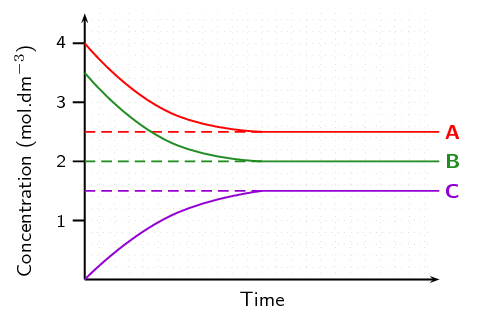[A] = $$\text{2,5}$$ $$\text{mol·dm^{-3}}$$

[B] = $$\text{2,0}$$ $$\text{mol·dm^{-3}}$$

[C] = $$\text{1,5}$$ $$\text{mol·dm^{-3}}$$

### Calculate $$\text{K}_{\text{c}}$$

$\text{K}_{\text{c}}=\frac{\text{[C]}}{\text{[A][B]}}=\frac{\text{1,5}}{(\text{2,5})(\text{2,0})} = \text{0,3}$

### Determine which reaction is exothermic and which is endothermic

The forward reaction has $$\Delta{H} > 0$$. This means that the forward reaction is endothermic. The reverse reaction must therefore be exothermic.

### Which reaction is favoured by an increase in temperature?

The endothermic reaction would be favoured by an increase in temperature (to lower the temperature). This is the forward reaction.

### How would [A], [B] and [C] change?

The forward reaction is favoured, therefore the equilibrium would shift to the right. This means that the reactant concentrations ([A] and [B]) would decrease and the product concentration ([C]) would increase.

## Equilibrium

Textbook Exercise 8.3

The following reaction has reached equilibrium in a closed container:

$\text{C}(\text{s}) + \text{H}_{2}\text{O}(\text{g}) \rightleftharpoons \text{CO}(\text{g}) + \text{H}_{2}(\text{g}) \quad \Delta{H} > 0$

The pressure of the system is then decreased. How will the concentration of the $$\text{H}_{2}$$(g) and the value of $$\text{K}_{\text{c}}$$ be affected when the new equilibrium is established? Assume that the temperature of the system remains unchanged.

 Hydrogen concentration $$\text{K}_{\text{c}}$$ (a) increases increases (b) increases stays the same (c) stays the same stays the same (d) decreases stays the same

(IEB Paper 2, 2004)

According to Le Chatelier's principle, the reaction that increases the number of gas molecules (and therefore the pressure) will be favoured. That is the forward reaction, and so the concentration of hydrogen will increase. Only temperature affects the value of $$\text{K}_{\text{c}}$$, therefore $$\text{K}_{\text{c}}$$ remains the same.

b) Hydrogen concentration increases and equilibrium constant stays the same

During a classroom experiment copper metal reacts with concentrated nitric acid to produce $$\text{NO}_{2}$$ gas.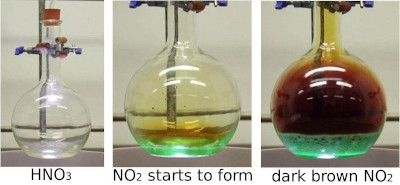The $$\text{NO}_{2}$$ is collected in a gas syringe.When enough gas has collected in the syringe, the delivery tube is clamped so that no gas can escape. The brown $$\text{NO}_{2}$$ gas collected reaches an equilibrium with colourless $$\text{N}_{2}\text{O}_{4}$$ gas as represented by the following equation:

$2\text{NO}_{2}(\text{g}) \rightleftharpoons \text{N}_{2}\text{O}_{4}(\text{g}), \quad \Delta H < 0$

Once this equilibrium has been established, there are $$\text{0,01}$$ $$\text{moles}$$ of $$\text{NO}_{2}$$ gas and $$\text{0,03}$$ $$\text{moles}$$ of $$\text{N}_{2}\text{O}_{4}$$ gas present in the syringe.

A learner, noticing that the colour of the gas mixture in the syringe is no longer changing, comments that all chemical reactions in the syringe must have stopped. Is this assumption correct? Explain.

No. The learner is not correct. A dynamic chemical equilibrium has been reached and the products are changing into reactants at the same rate as reactants are changing into products. This results in no colour change being observed in the syringe.

The gas in the syringe is cooled. The volume of the gas is kept constant during the cooling process. Will the gas be lighter or darker at the lower temperature? Explain your answer.

The forward reaction is exothermic ($$\Delta H < 0$$). A decrease in temperature will favour the forward reaction. $$\text{N}_{2}\text{O}_{4}(\text{g})$$ is colourless, therefore the gas will be lighter at the lower temperature.

The volume of the syringe is now reduced (at constant temperature) to $$\text{75}$$ $$\text{cm^{3}}$$ by pushing the plunger in and holding it in the new position. There are $$\text{0,032}$$ $$\text{moles}$$ of $$\text{N}_{2}\text{O}_{4}$$ gas present once the equilibrium has been re-established at the reduced volume ($$\text{75}$$ $$\text{cm^{3}}$$). Calculate the value of the equilibrium constant for this equilibrium.

(IEB Paper 2, 2004)

The volume is reduced, so the pressure is increased. This means that the foward reaction will be favoured to reduce the number of gas molecules.

There are $$\text{0,01}$$ moles of $$\text{NO}_{2}$$ and $$\text{0,03}$$ moles of $$\text{N}_{2}\text{O}_{4}$$ initially.

For every 2x moles of $$\text{NO}_{2}$$ used, 1x moles of $$\text{N}_{2}\text{O}_{4}$$ are produced.

 Reaction $$2\text{NO}_{2}(\text{g})$$ $$1\text{N}_{2}\text{O}_{4}(\text{g})$$ Initial quantity (mol) $$\text{0,01}$$ $$\text{0,03}$$ Change (mol) -2x +x Equilibrium quantity (mol) $$\text{0,01}$$-2x $$\text{0,03}$$+x Equilibrium concentration ($$\text{mol·dm^{-3}}$$)

There are $$\text{0,032}$$ $$\text{mol}$$ $$\text{N}_{2}\text{O}_{4}$$ at the new equilibrium.

Therefore $$\text{0,03}$$ $$\text{mol}$$ + x = $$\text{0,032}$$ $$\text{mol}$$

x = $$\text{0,032}$$ - $$\text{0,03}$$ $$\text{mol}$$ = $$\text{0,002}$$ $$\text{mol}$$

n($$\text{NO}_{2}$$) at equilibrium = $$\text{0,01}$$ $$\text{mol}$$ - $$\text{2}$$ $$\times$$ $$\text{0,002}$$ $$\text{mol}$$ = $$\text{0,006}$$ $$\text{mol}$$

C($$\text{NO}_{2}$$) at equilibrium = $$\dfrac{\text{0,006}\text{ mol}}{\text{0,075}\text{ dm^{3}}}$$ = $$\text{0,08}$$ $$\text{mol·dm^{-3}}$$

C($$\text{N}_{2}\text{O}_{4}$$) at equilibrium = $$\dfrac{\text{0,032}\text{ mol}}{\text{0,075}\text{ dm^{3}}}$$ = $$\text{0,43}$$ $$\text{mol·dm^{-3}}$$

 Reaction $$2\text{NO}_{2}(\text{g})$$ $$1\text{N}_{2}\text{O}_{4}(\text{g})$$ Initial quantity (mol) $$\text{0,01}$$ 0.03 Change (mol) -2x +x Equilibrium quantity (mol) $$\text{0,01}$$-2x $$\text{0,03}$$+x Equilibrium concentration ($$\text{mol·dm^{-3}}$$) $$\text{0,08}$$ $$\text{0,43}$$

$$\text{K}_{\text{c}}=\dfrac{\text{[N}_{2}\text{O}_{4}\text{]}}{\text{[NO}_{2}\text{]}^{2}}=\dfrac{\text{0,43}}{(\text{0,08})^{2}}=\text{67,19}$$

Gases X and Y are pumped into a $$\text{2}$$ $$\text{dm^{3}}$$ container. When the container is sealed, $$\text{4}$$ $$\text{moles}$$ of gas X and $$\text{4}$$ $$\text{moles}$$ of gas Y are present. The following equilibrium is established:

$$2\text{X}(\text{g}) + 3\text{Y}(\text{g})$$ $$\rightleftharpoons$$ $$\text{X}_{2}\text{Y}_{3}(\text{g})$$

The graph below shows the number of moles of gas X and gas $$\text{X}_{2}\text{Y}_{3}$$ that are present from the time the container is sealed.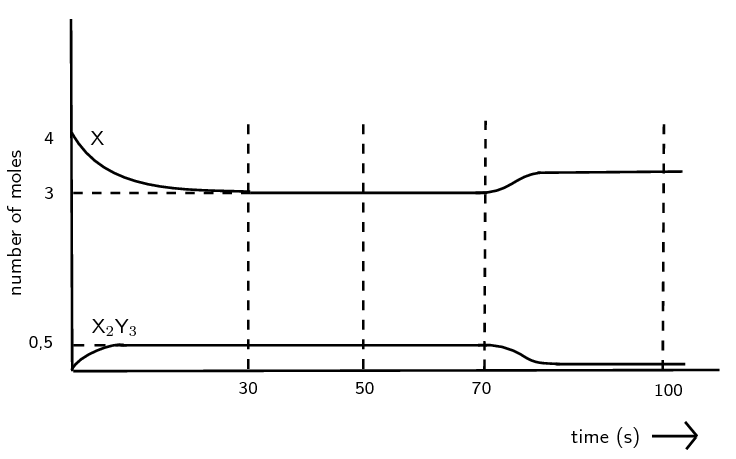How many moles of gas $$\text{X}_{2}\text{Y}_{3}$$ are formed by the time the reaction reaches equilibrium at $$\text{30}$$ $$\text{seconds}$$?

From the graph: $$\text{0,5}$$ $$\text{mol}$$

Calculate the value of the equilibrium constant at t = $$\text{50}$$ $$\text{s}$$.

Draw up a RICE table.

 Reaction $$2\text{X}(\text{g})$$ $$3\text{Y}(\text{g})$$ $$\text{X}_{2}\text{Y}_{3}(\text{g})$$ Initial quantity (mol) $$\text{4}$$ $$\text{4}$$ $$\text{0}$$ Change (mol) -2x -3x +x Equilibrium quantity (mol) $$\text{4}$$ - 2x $$\text{4}$$ - 3x $$\text{0}$$ + x Equilibrium concentration ($$\text{mol·dm^{-3}}$$)

There are $$\text{0,5}$$ $$\text{mol}$$ of $$\text{X}$$ present at equilibrium.

Therefore x = $$\text{0,5}$$ $$\text{mol}$$

From the graph n($$\text{X}$$) = $$\text{3}$$ $$\text{mol}$$

n($$\text{Y}$$) = $$\text{4}$$ - $$\text{3}$$ x $$\text{0,5}$$ $$\text{mol}$$ = $$\text{2,5}$$ $$\text{mol}$$

 Reaction $$2\text{X}(\text{g})$$ $$3\text{Y}(\text{g})$$ $$\text{X}_{2}\text{Y}_{3}(\text{g})$$ Initial quantity (mol) $$\text{4}$$ $$\text{4}$$ $$\text{0}$$ Change (mol) $$-\text{1}$$ $$-\text{1,5}$$ $$\text{+0,5}$$ Equilibrium quantity (mol) $$\text{3}$$ $$\text{2,5}$$ $$\text{0,5}$$ Equilibrium concentration ($$\text{mol·dm^{-3}}$$)

C($$\text{X}$$) = $$\dfrac{\text{3}\text{ mol}}{\text{2}\text{ dm^{3}}} =$$$$\text{1,5}$$ $$\text{mol·dm^{-3}}$$

C($$\text{Y}$$) = $$\dfrac{\text{2,5}\text{ mol}}{\text{2}\text{ dm^{3}}} =$$$$\text{1,25}$$ $$\text{mol·dm^{-3}}$$

C($$\text{X}_{2}\text{Y}_{3}$$) = $$\dfrac{\text{0,5}\text{ mol}}{\text{2}\text{ dm^{3}}} =$$$$\text{0,25}$$ $$\text{mol·dm^{-3}}$$

 Reaction $$2\text{X}(\text{g})$$ $$3\text{Y}(\text{g})$$ $$\text{X}_{2}\text{Y}_{3}(\text{g})$$ Initial quantity (mol) $$\text{4}$$ $$\text{4}$$ $$\text{0}$$ Change (mol) $$-\text{1}$$ $$-\text{1,5}$$ $$\text{+0,5}$$ Equilibrium quantity (mol) $$\text{3}$$ $$\text{2,5}$$ $$\text{0,5}$$ Equilibrium concentration ($$\text{mol·dm^{-3}}$$) $$\text{1,5}$$ $$\text{1,25}$$ $$\text{0,25}$$

$${K}_{c}=\dfrac{\text{[X}_{2}\text{Y}_{3}\text{]}}{\text{[X]}^{2}\text{[Y]}^{3}}=\dfrac{\text{0,25}}{(\text{1,5})^{2}(\text{1,25})^{3}}=\text{0,057}$$

At $$\text{70}$$ $$\text{s}$$ the temperature is increased. Is the forward reaction endothermic or exothermic? Explain in terms of Le Chatelier's Principle.

Exothermic. The amount of product decreases (and amount of reactants increases) when the temperature is increased indicating that the reverse reaction is favoured. Le Chatelier's principle states that an increase in temperature will favour the endothermic reaction (cooling the reaction vessel). Therefore the reverse reaction must be endothermic and the forward reaction is exothermic.

How will this increase in temperature affect the value of the equilibrium constant?

A change in temperature is the only thing that affects the equilibrium constant. The amount of product decreased and the amount of reactants increased, therefore the equilibrium constant will decrease.

Consider the following hypothetical reaction that takes place in a closed $$\text{2}$$ $$\text{dm^{3}}$$ flask at $$\text{298}$$ $$\text{K}$$.

$$\text{A}_{2}(\text{g}) + 2\text{B}_{2}(\text{g})$$ $$\rightleftharpoons$$ $$2\text{AB}_{2}(\text{g})$$

The graph beneath represents the change in the number of moles of each gas in the flask over a period of $$\text{20}$$ $$\text{minutes}$$.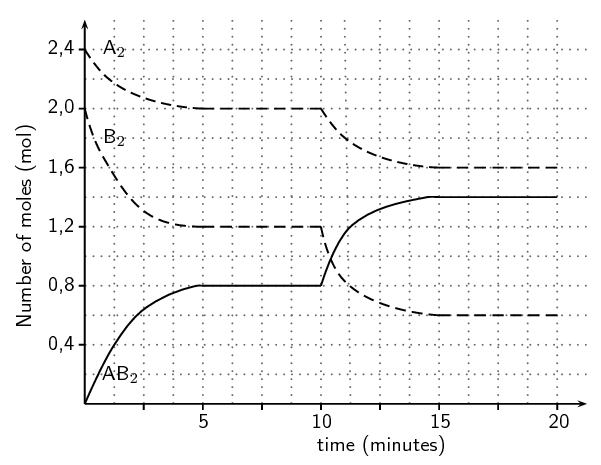State how long (in minutes) it took for the reaction to reach equilibrium for the first time.

The number of moles of each reactant and the product is constant (have reached equilibrium) after t = $$\text{5}$$ $$\text{minutes}$$

Write down an expression for the equilibrium constant, $$\text{K}_{\text{c}}$$, for this particular reaction.

$$\text{K}_{\text{c}} = \dfrac{\text{[AB}_{2}{\text{]}}^{2}}{\text{[A}_{2}{\text{][B}}_{2}{\text{]}}^{2}}$$

Calculate the concentration of each of the reactants and the product using figures from the graph between $$\text{5}$$ $$\text{minutes}$$ and $$\text{10}$$ $$\text{minutes}$$ and hence calculate the equilibrium constant $$\text{K}_{\text{c}}$$ for this reaction at $$\text{298}$$ $$\text{K}$$

The concentration values are read off the graph.

C($$\text{A}_{2}$$) $$= \dfrac{\text{2,0}\text{ mol}}{\text{2}\text{ dm^{3}}} =$$ $$\text{1,0}$$ $$\text{mol·dm^{-3}}$$

C($$\text{B}_{2}$$) $$= \dfrac{\text{1,2}\text{ mol}}{\text{2}\text{ dm^{3}}} =$$ $$\text{0,6}$$ $$\text{mol·dm^{-3}}$$

C($$\text{AB}_{2}$$) $$= \dfrac{\text{0,8}\text{ mol}}{\text{2}\text{ dm^{3}}} =$$ $$\text{0,4}$$ $$\text{mol·dm^{-3}}$$

$$\text{K}_{\text{c}} = \dfrac{\text{[AB}_{2}{\text{]}}^{2}}{\text{[A}_{2}{\text{][B}_{2}{\text{]}}^{2}}} = \dfrac{\text{0,4}^{2}}{(\text{1,0})(\text{0,6})^{2}} =$$ $$\text{0,44}$$

State what a low value of $$\text{K}_{\text{c}}$$ indicates about the yield of product for a reaction.

A value of $$\text{K}_{\text{c}}$$ between $$\text{0}$$ and $$\text{1}$$ indicates that the equilibrium lies to the left. Therefore the yield of product is low (more reactants than products).

Why is it not possible to calculate $$\text{K}_{\text{c}}$$ using figures from the graph during the first $$\text{5}$$ $$\text{minutes}$$

$$\text{K}_{\text{c}}$$ is the equilibrium constant, therefore it cannot be calculated except when the reaction is in equilibrium. During the first $$\text{5}$$ $$\text{minutes}$$ the reaction is not in equilibrium.

State Le Chatelier's principle.

When an external stress (change in pressure, temperature or concentration) is applied to a system in chemical equilibrium, the equilibrium will change in such a way as to reduce the effect of the stress.

At $$\text{10}$$ $$\text{minutes}$$ the temperature of the flask was increased. Using Le Chatelier's principle, determine if the production of $$\text{AB}_{2}$$ is exothermic or endothermic?

$$\text{AB}_{2}$$ is produced through the forward reaction. Le Chatelier's principle states that if the temperature is increased the equilibrium will change to decrease the temperature of the vessel. That is the reaction that takes in heat (endothermic) will be favoured.

The number of moles of the reactants decreases after the temperature increase, while the number of moles of the product increases. Therefore the temperature increase favours the forward reaction. This means that the forward reaction is endothermic.

How would the equilibrium constant be affected by each of the following changes:

(state either increase, decrease or no effect)

Increasing the pressure of the flask with no change to temperature.

no effect

no effect

Increasing the temperature of the flask.

increase

### Industrial applications (ESCNX)

In industrial processes, it is important to get the product as quickly and as efficiently as possible. The less expensive the process the better.

The Haber process is a good example of an industrial process which uses the equilibrium principles that have been discussed. The equation for the process is as follows:

$\text{N}_{2}(\text{g}) + 3\text{H}_{2}(\text{g}) \rightleftharpoons 2\text{NH}_{3}(\text{g}) + \text{energy}$

Since the forward reaction is exothermic, to produce a lot of product and favour the forward reaction the system needs to be colder. However, cooling a system slows down all chemical reactions and so the system can't be too cold. This process is carried out at a much higher temperature to ensure the speed of production.

Because high temperature favours the reverse reaction, the $$\text{NH}_{3}$$ product is actually removed as it is made (product concentration decreased) to prevent ammonia being used in the reverse reaction. The decrease of product concentration favours the forward reaction.

High pressure is also used to ensure faster reaction time and to favour the production of $$\text{NH}_{3}$$. The forward reaction is favoured by higher pressures because there are $$\text{2}$$ gas molecules of product for every $$\text{4}$$ gas molecules of reactant.

Refer to Chapter 14 for more information on the Haber process and other industrial applications.

## Applying equilibrium principles

Textbook Exercise 8.4

Look at the values of $$\text{K}_{\text{c}}$$ calculated for the Haber process reaction at different temperatures, and then answer the questions that follow:

 $$T\left(℃\right)$$ $$\text{K}_{\text{c}}$$ $$\text{25}$$ $$\text{6,4} \times \text{10}^{\text{2}}$$ $$\text{200}$$ $$\text{4,4} \times \text{10}^{-\text{1}}$$ $$\text{300}$$ $$\text{4,3} \times \text{10}^{-\text{3}}$$ $$\text{400}$$ $$\text{1,6} \times \text{10}^{-\text{4}}$$ $$\text{500}$$ $$\text{1,5} \times \text{10}^{-\text{5}}$$

What happens to the value of $$\text{K}_{\text{c}}$$ as the temperature increases?

The value of $$\text{K}_{\text{c}}$$ decreases

Which reaction is being favoured when the temperature is $$\text{300}$$ $$\text{℃}$$?

A value of $$\text{K}_{\text{c}}$$ between 0 and 1 means that there are more reactants than products. Therefore the reverse reaction is favoured.

According to this table, which temperature would be best if you wanted to produce as much ammonia as possible? Explain.

$$\text{25}$$ $$\text{℃}$$. At this temperature the forward reaction is favoured and so the the maximum yield of ammonia is achieved.